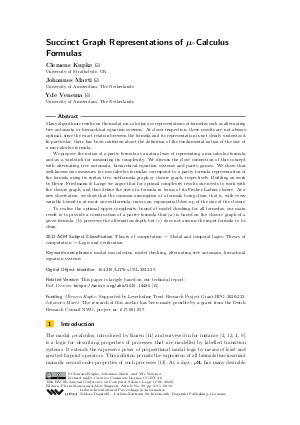Document# Succinct Graph Representations of μ-Calculus Formulas

### Authors Clemens Kupke, Johannes Marti, Yde Venema## File

LIPIcs.CSL.2022.29.pdf
• Filesize: 0.83 MB
• 18 pages

## Cite As

Clemens Kupke, Johannes Marti, and Yde Venema. Succinct Graph Representations of μ-Calculus Formulas. In 30th EACSL Annual Conference on Computer Science Logic (CSL 2022). Leibniz International Proceedings in Informatics (LIPIcs), Volume 216, pp. 29:1-29:18, Schloss Dagstuhl - Leibniz-Zentrum für Informatik (2022)
https://doi.org/10.4230/LIPIcs.CSL.2022.29

## Abstract

Many algorithmic results on the modal mu-calculus use representations of formulas such as alternating tree automata or hierarchical equation systems. At closer inspection, these results are not always optimal, since the exact relation between the formula and its representation is not clearly understood. In particular, there has been confusion about the definition of the fundamental notion of the size of a mu-calculus formula. We propose the notion of a parity formula as a natural way of representing a mu-calculus formula, and as a yardstick for measuring its complexity. We discuss the close connection of this concept with alternating tree automata, hierarchical equation systems and parity games. We show that well-known size measures for mu-calculus formulas correspond to a parity formula representation of the formula using its syntax tree, subformula graph or closure graph, respectively. Building on work by Bruse, Friedmann & Lange we argue that for optimal complexity results one needs to work with the closure graph, and thus define the size of a formula in terms of its Fischer-Ladner closure. As a new observation, we show that the common assumption of a formula being clean, that is, with every variable bound in at most one subformula, incurs an exponential blow-up of the size of the closure. To realise the optimal upper complexity bound of model checking for all formulas, our main result is to provide a construction of a parity formula that (a) is based on the closure graph of a given formula, (b) preserves the alternation-depth but (c) does not assume the input formula to be clean.

## Subject Classification

##### ACM Subject Classification
• Theory of computation → Modal and temporal logics
• Theory of computation → Logic and verification
##### Keywords
• modal mu-calculus
• model checking
• alternating tree automata
• hierachical equation systems

## Metrics

• Access Statistics
• Total Accesses (updated on a weekly basis)
0

## References

1. B. Afshari and G. Leigh. Cut-free completeness for modal mu-calculus. In Proceedings of the 32nd Annual ACM/IEEE Symposium on Logic In Computer Science (LICS'17), pages 1-12. IEEE Computer Society, 2017.2. A. Arnold and D. Niwiński. Rudiments of μ-calculus, volume 146 of Studies in Logic and the Foundations of Mathematics. North-Holland Publishing Co., Amsterdam, 2001.3. D. Baelde, A. Doumane, and A. Saurin. Infinitary proof theory: the multiplicative additive case. In J.-M. Talbot and L. Regnier, editors, Proceedings of the 25th EACSL Annual Conference on Computer Science Logic, CSL 2016, volume 62 of LIPIcs. Schloss Dagstuhl - Leibniz-Zentrum für Informatik, 2016.4. J. Bradfield and C. Stirling. Modal μ-calculi. In J. van Benthem, P. Blackburn, and F. Wolter, editors, Handbook of Modal Logic, pages 721-756. Elsevier, 2006.5. J.C. Bradfield and I. Walukiewicz. The mu-calculus and model checking. In E. M. Clarke, Th.A. Henzinger, H. Veith, and R. Bloem, editors, Handbook of Model Checking, pages 871-919. Springer, 2018. URL: https://doi.org/10.1007/978-3-319-10575-8_26.
6. F. Bruse, O. Friedmann, and M. Lange. On guarded transformation in the modal μ-calculus. Logic Journal of the IGPL, 23(2):194-216, 2015.7. C.S. Calude, S. Jain, B. Khoussainov, W. Li, and F. Stephan. Deciding parity games in quasipolynomial time. In H. Hatami, P. McKenzie, and V. King, editors, Proceedings of the 49th Annual ACM SIGACT Symposium on Theory of Computing, (STOC 2017), pages 252-263, 2017.8. G. D'Agostino and M. Hollenberg. Logical questions concerning the μ-calculus. Journal of Symbolic Logic, 65:310-332, 2000.9. S. Demri, V. Goranko, and M. Lange. Temporal Logics in Computer Science: Finite-State Systems. Cambridge Tracts in Theoretical Computer Science. Cambridge University Press, 2016.10. E.A. Emerson and C.S. Jutla. Tree automata, mu-calculus and determinacy (extended abstract). In Proceedings of the 32nd Symposium on the Foundations of Computer Science, pages 368-377. IEEE Computer Society Press, 1991.11. G. Fontaine and Y. Venema. Some model theory for the modal mu-calculus: syntactic characterizations of semantic properties. Logical Methods in Computer Science, 14(1), 2018.12. E. Grädel, W. Thomas, and T. Wilke, editors. Automata, Logic, and Infinite Games, volume 2500 of LNCS. Springer, 2002.13. D. Janin and I. Walukiewicz. On the expressive completeness of the propositional μ-calculus w.r.t. monadic second-order logic. In Proceedings of the Seventh International Conference on Concurrency Theory, CONCUR '96, volume 1119 of LNCS, pages 263-277, 1996.14. D. Kozen. Results on the propositional μ-calculus. Theoretical Computer Science, 27:333-354, 1983.15. C. Kupke, J. Marti, and Y. Venema. Size matters in the modal μ-calculus, 2020. URL: http://arxiv.org/abs/2010.14430.
16. D. Niwiński. On fixed point clones. In L. Kott, editor, Proceedings of the 13th International Colloquium on Automata, Languages and Programming (ICALP 13), volume 226 of LNCS, pages 464-473, 1986.17. D. Niwiński. Fixed point characterization of infinite behavior of finite-state systems. Theoretical Computer Science, 189:1-69, 1997.18. H. Seidl and A. Neumann. On guarding nested fixpoints. In Proceedings of the 13th EACSL Annual Conference on Computer Science Logic, CSL '99, pages 484-498, 1999.19. I. Walukiewicz. Completeness of Kozen’s axiomatisation of the propositional μ-calculus. Information and Computation, 157:142-182, 2000.20. T. Wilke. Alternating tree automata, parity games, and modal μ-calculus. Bulletin of the Belgian Mathematical Society, 8:359-391, 2001.X

Feedback for Dagstuhl Publishing